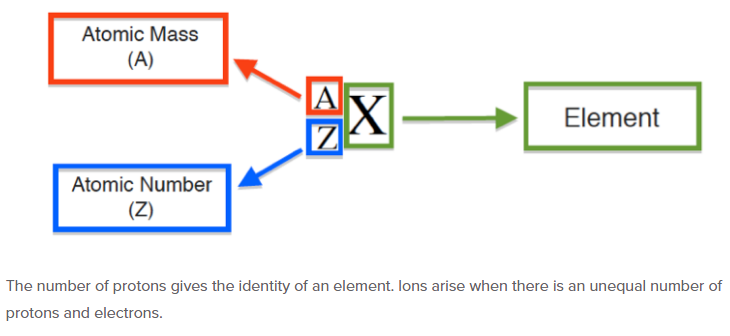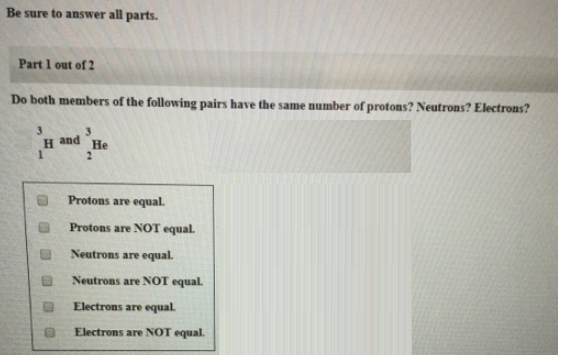# Problem: Be sure to answer all parts.Part 1 of 2Do both members of the following pairs have the same number of protons? Neutrons? Electrons? 3H1 and 3He2             (i) Protons are equal. (ii) Protons are NOT equal. (iii) Neutrons are equal. (iv) Neutrons are NOT equal. (v) Electrons are equal. (vi) Electrons are NOT equal.

###### FREE Expert Solution(i) Protons are equal. (False, atomic number = proton)

93% (51 ratings)###### Problem Details

Be sure to answer all parts.

Part 1 of 2

Do both members of the following pairs have the same number of protons? Neutrons? Electrons?

3H1 and 3He2

(i) Protons are equal.

(ii) Protons are NOT equal.

(iii) Neutrons are equal.

(iv) Neutrons are NOT equal.

(v) Electrons are equal.

(vi) Electrons are NOT equal.Frequently Asked Questions

What scientific concept do you need to know in order to solve this problem?

Our tutors have indicated that to solve this problem you will need to apply the Subatomic Particles concept. You can view video lessons to learn Subatomic Particles. Or if you need more Subatomic Particles practice, you can also practice Subatomic Particles practice problems.

What professor is this problem relevant for?

Based on our data, we think this problem is relevant for Professor Roy's class at GMU.